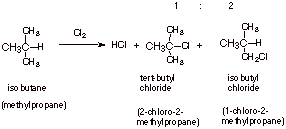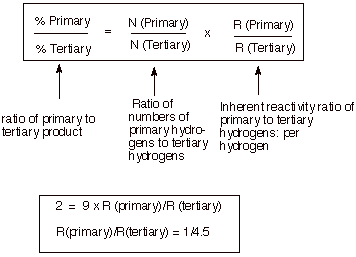Statistical Effects Can Be Important in Unselective Reactions Like Chlorinationq      The chlorination of alkanes is quite unselective, but the inherent order of reactivity is tertiary hydrogens greater than secondary, greater than primary (the magnitudes of the preferences, however, are small). However, note that in the chlorination of isobutane, the amount of the product corresponding to substitution at the primary hydrogen actually exceeds the amount of product corresponding to substitution at the tertiary hydrogen. The reason for this is a statistical (probability) effect.

q      Since there are nine times as many primary hydrogens (see three methyl groups)  as tertiary, the probability that a chlorine atom will encounter (collide with) a primary hydrogen is statistically nine times as great as with a tertiary hydrogen. Since the inherent preference for tertiary over primary is less than the factor of nine, there is slightly more primary chloride produced than tertiary chloride.q      Note that the tertiary hydrogens are, per hydrogen, more reactive than the primary hydrogens, per hydrogen, by a factor of 4.5.